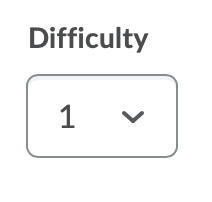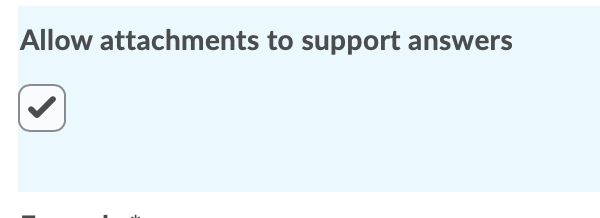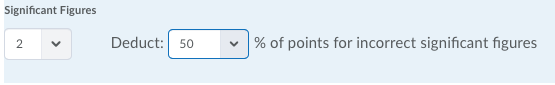# Create Significant Figures Questions in the Question Library

Significant figures questions require respondents to answer in scientific notation and provide solutions that contain a specified number of significant figures. Math and science courses commonly use this question type. You can ensure each respondent receives a unique question by including variables enclosed with curly braces that randomly generate scientific notations within the problem.

## Understanding Arithmetic and Significant Figures Question Components

In significant figures questions, you can select a percentage of the answer’s score to deduct for including incorrect significant figures in a response.

Use tolerance levels to accept near-accurate, estimated, or rounded answers.

Watch how to create significant figures question

Note: For a secure and consistent editing experience, JavaScript and non-standard HTML will automatically be filtered out. If your existing questions contain JavaScript or non-standard HTML, the questions continue to display as intended until an instructor edits and saves the quizzes, which removes the JavaScript and non-standard HTML.

1. On the nav bar, select the Activities menu, then click either Quizzes, Surveys, or Self Assessments. In the example below, we selected Quizzes.2. From the tool navigation, click Question Library.3. Select New.4. Select the question type: Significant Figures.
5. Optional: Title Note: We recommend leaving the Title field blank. The Question Text will become the title by default, allowing you to see the question without having to click to open it.6. Required: Points7. Optional: Difficulty Level8. Required: Question Text:  Enclose all variables with curly braces if you want to generate random numbers. To display the value of a variable, ensure that the variable is enclosed in curly braces. For example:What is the sum of {x} and {y}?
Note: The Graphical Equation editor can be especially helpful if the question contains a complicated formula. If you are working on a device with a small screen, you may have to click the ellipses for the Graphical Equation menu to appear.9. Optional: Allow attachments to support answers:  Check the box to allow students to attach files with evidence supporting their answer.• When students are reviewing their quiz submissions, if they are allowed to view their quiz responses, they can view their uploaded attachments.
• The maximum file size for a single file or embedded image attached to a quiz response is set to 102400 KB (100MB). The maximum file size for all files attached or embedded in a single quiz question response is set to 102400 KB (100MB).
10. Enter the solution’s formula, setting variables inside curly braces. See Types of Supported Enumerations in the Formula Field.11. To verify your formula before sharing it with students, click Test.
12. Use the Significant Figures drop-down menu to define the number of significant figures the correct answer should contain. If you want to give partial credit to a student who provides the correct answer but uses the incorrect number of significant figures, use the Worth drop-down menu to tell D2L what percentage of the question’s points to deduct. Note: If you require a specific number of significant figures in the answer, we suggest explaining that in your question or in the quiz instructions.13. To accept near-accurate, estimated, and/or rounded answers, enter a Tolerance level. Learn about Understanding Quiz Answer Rounding Rules:
14. Use this option if the correct answer should include a unit of measurement (mm, cm, grams, inches, etc.):
15. Select the Evaluation Option. There are three possible grading options:
16. In the Variables area, do the following:
• Create any variables you want to use with your question by giving the variable a Name, a minimum value in the Min field, and a maximum value in the Max field.  Note: The name should exactly match the corresponding variable in your formula.
• To set the system’s incrementing steps as it generates numbers from the range set by the Min and Max fields, enter a number in the Step field.  Assign a range from which D2L can randomly assign a value to that variable. If you do not want D2L to randomly assign a value, then set the same number in both the Min and Max fields.17. Optional: Add or remove Variables, as needed, to match the number of variables in the formula. Add a variable by clicking the Add Variable link. Delete a variable by selecting the Remove Variable (trash can icon).
18. Optional: To add overall feedback for the question, select Add Feedback. Learn about Quiz Question Feedback.
19. Optional: To add a hint to the question, select Add Hint. Learn about Quiz Question Hints.